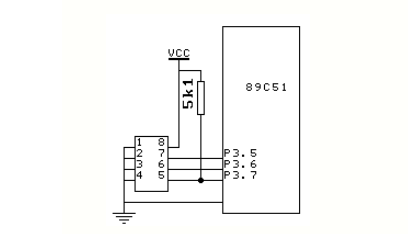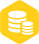# 如何使用C语言实现51单片机模拟I2C总线

1.1w人已加入

### 描述

电路原理图EEPROM为ATMEL公司的AT24C01A。单片机为ATMEL公司的AT89C51。

软件说明

C语言为Franklin C V3.2。将源程序另存为testi2c.c，用命令

C51 testi2c.c

L51 TESTI2C.OBJ

OHS51 TESTI2C

编译，连接，得到TESTI2C.HEX文件，即可由编程器读入并进行写片，实验。

3．源程序

#include 《reg51.h》

#include 《intrins.h》

#define uchar unsigned char

#define uint unsigned int

#define Hidden 0x0e /*显示器的消隐码*/

/*有关全局变量*/

sbit Sda= P3^7; /*串行数据*/

sbit Scl= P3^6; /*串行时钟*/

sbit WP= P3^5; /*硬件写保护*/

void mDelay（uchar j）

{ uint i;

for（;j》0;j--）

{ for（i=0;i《125;i--）

{;}

}

}

/*发送起始条件*/

void Start（void） /*起始条件*/

{

Sda=1;

Scl=1;

_nop_ （）;

_nop_ （）;

_nop_ （）;

_nop_ （）;

Sda=0;

_nop_ （）;

_nop_ （）;

_nop_ （）;

_nop_ （）;

}

void Stop（void） /*停止条件*/

{

Sda=0;

Scl=1;

_nop_ （）;

_nop_ （）;

_nop_ （）;

_nop_ （）;

Sda=1;

_nop_ （）;

_nop_ （）;

_nop_ （）;

_nop_ （）;

}

void Ack（void） /*应答位*/

{

Sda=0;

_nop_ （）;

_nop_ （）;

_nop_ （）;

_nop_ （）;

Scl=1;

_nop_ （）;

_nop_ （）;

_nop_ （）;

_nop_ （）;

Scl=0;

}

void NoAck（void） /*反向应答位*/

{

Sda=1;

_nop_ （）;

_nop_ （）;

_nop_ （）;

_nop_ （）;

Scl=1;

_nop_ （）;

_nop_ （）;

_nop_ （）;

_nop_ （）;

Scl=0;

}

void Send（uchar Data） /*发送数据子程序，Data为要求发送的数据*/

{

uchar BitCounter=8; /*位数控制*/

uchar temp; /*中间变量控制*/

do

{

temp=Data;

Scl=0;

_nop_ （）;

_nop_ （）;

_nop_ （）;

_nop_ （）;

if（（temp&0x80）==0x80）/* 如果最高位是1*/

Sda=1;

else

Sda=0;

Scl=1;

temp=Data《《1; /*RLC*/

Data=temp;

BitCounter--;

}while（BitCounter）;

Scl=0;

}

{

uchar temp=0;

uchar temp1=0;

uchar BitCounter=8;

Sda=1;

do{

Scl=0;

_nop_ （）;

_nop_ （）;

_nop_ （）;

_nop_ （）;

Scl=1;

_nop_ （）;

_nop_ （）;

_nop_ （）;

_nop_ （）;

if（Sda） /*如果Sda=1;*/

temp=temp|0x01; /*temp的最低位置1*/

else

temp=temp&0xfe; /*否则temp的最低位清0*/

if（BitCounter-1）

{ temp1=temp《《1;

temp=temp1;

}

BitCounter--;

}while（BitCounter）;

return（temp）;

}

{

uchar i;

uchar *PData;

PData=Data;

for（i=0;i《Num;i++）

{

Start（）; /*发送启动信号*/

Send（0xa0）; /*发送SLA+W*/

Ack（）;

Ack（）;

Send（*（PData+i））;

Ack（）;

Stop（）;

mDelay（20）;

}

}

{

uchar i;

uchar *PData;

PData=Data;

for（i=0;i《Num;i++）

{

Start（）;

Send（0xa0）;

Ack（）;

Ack（）;

Start（）;

Send（0xa1）;

Ack（）;

Scl=0;

NoAck（）;

Stop（）;

}

}

void main（）

{

uchar Number［4］={1，2，3，4};

WP= 1;

WrToROM（Number，4，4）; /*将初始化后的数值写入EEPROM*/

mDelay（20）;

Number［0］=0;

Number［1］=0;

Number［2］=0;

Number［3］=0; /*将数组中的值清掉，以验证读出的数是否正确*/

RdFromROM（Number，4，4）;

}×
20